Request a call back

how to derive mirror equation?
Asked by Akshatha Chandrashekar | 02 Oct, 2013, 05:33: PMExpert Answer

Mirror Formula (Concave Mirror)

Mirror formula is the relationship between object distance (u), image distance (v) and focal length.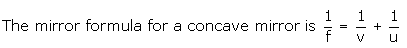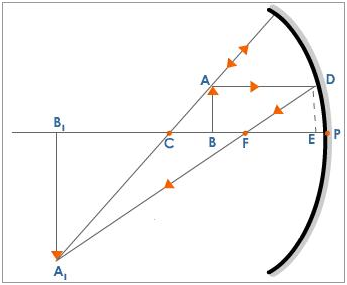## Derivation

The figure shows an object AB at a distance 'u' from the pole of a concave mirror. The image A1B1 is formed at a distance 'v' from the mirror. The position of the image is obtained by drawing a ray diagram.

Consider the D A1CB1 and D ACB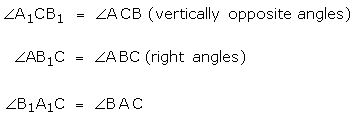[when two angles of D A1CB1 and D ACB are equal then the third angle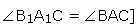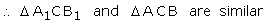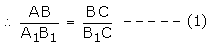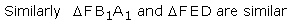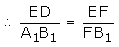But ED = AB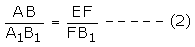From equations (1) and (2)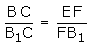If D is very close to P then EF = PF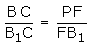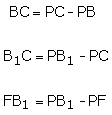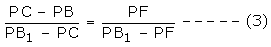But PC = R, PB = u, PB 1 = v, PF = f

By sign convention

PC = -R, PB = -u, PF = -f and PB 1 = -v

Equation (3) can be written as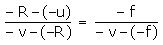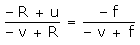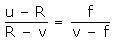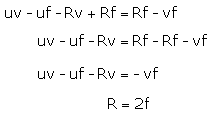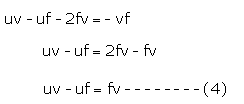Dividing equation (4) throughout by uvf we get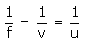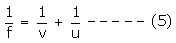Hence, equation (5) gives the mirror formula.

Answered by Faiza Lambe | 02 Oct, 2013, 07:07: PM

## Concept Videos

CBSE 12-science - Physics
Asked by bmahalik21 | 04 Mar, 2023, 07:03: PMANSWERED BY EXPERT
CBSE 12-science - Physics
Asked by gazanfarali5782 | 09 Jan, 2023, 03:21: PMANSWERED BY EXPERT
CBSE 12-science - Physics
Asked by zahirqk | 08 Jan, 2023, 09:50: AMANSWERED BY EXPERT
CBSE 12-science - Physics
Optical instrumentAsked by tannudahiya80536 | 20 Dec, 2022, 01:13: PMANSWERED BY EXPERT
CBSE 12-science - PhysicsANSWERED BY EXPERT
CBSE 12-science - Physics
soleAsked by hanskaraja | 05 Aug, 2022, 11:20: PMANSWERED BY EXPERT
CBSE 12-science - PhysicsANSWERED BY EXPERTANSWERED BY EXPERTANSWERED BY EXPERTANSWERED BY EXPERT